SEARCH HOMEMath Central Quandaries & QueriesQuestion from Leah, a student: I am having trouble finding the equation for this problem.: What is the volume of a cube with diagonals of length 6 sqrt(3) cm? answer: (BLANK) cm^3Leah, we have two responses for you below.

Hi Leah,

This is a fun problem because you get to use Pythagoras' theorem twice.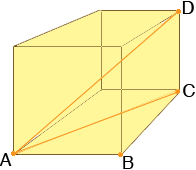Suppose that the length of a side of the cube is a cm then |AB| = |BC| = a. Since angle ABC is a right angle you can use Pythagoras' theorem to find the length of CA in terms of a. Also angle ACD is a right angle and you know the lengths of DC and CA so you can use Pythagoras' theorem again to find the length of DA in terms of a. But the length of DA is 6 √3 cm and hence you have an equation that you can solve for a.

I hope this helps,
Penny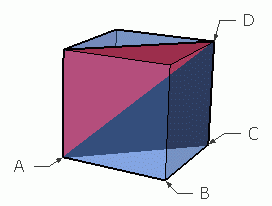Hi Leah.

Let d = the length of diagonal |AD| and
let a = the length of each side of the cube.

Pythagorus #1 in triangle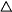ABC: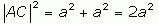Pythagorus #2 in triangleACD: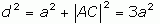Now we can solve for a in terms of d: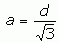This means that the volume V is: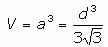Stephen La Rocque.Math Central is supported by the University of Regina and The Pacific Institute for the Mathematical Sciences.